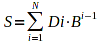# FFFFFFFF

What is FFFFFFFF

HP Probook 4510s Oops

Unlock x220l usb stick

## What is FFFFFFFF?

### Most of all, FFFFFFFF is a number in hexadecimal notation

FFFFFFFF is the biggest 32-bit number in hexadecimal (or hex) notation. The hexadecimal notation is a way of representing base 16 numbers. The digits 0 to 9 are written as in the decimal numbers, while the digits 10 to 15 are written as A to F. The number is the sum of all the digits multiplied by 16 powered to the subtraction of the position of the digit from right to left and 1.where S is the resulting number, N is the number of digits of the number, i is the position from right to left of the digit Di in the number, B is the base, which is 16 in our case.

If we calculate the number in decimal, FFFFFFFF in decimal is 4294967295. In octal FFFFFFFF is 37777777777 or 037777777777. In binary FFFFFFFF is 11111111111111111111111111111111 or 0b11111111111111111111111111111111 . In quaternary base FFFFFFFF is 3333333333333333. The above was true if we interpret FFFFFFFF as unsigned integer. If we interpret FFFFFFFF as a signed integer and the maximum bits of the number are 32, then FFFFFFFF is -1.

If we increase FFFFFFFF by 1, we get 100000000, it it was possible or overflow 32 bits, and we get 00000000 if it was not possible to overflow 32 bits. If we decrease FFFFFFFF by 1, we get FFFFFFFE.

We can use the hexadecimal representation to conduct operations on binary numbers. If we use 32-bit registers to calculate the operations, x AND FFFFFFFF = x, x OR FFFFFFFF = FFFFFFFF, and, if we interpret the numbers as signed integers, x XOR FFFFFFFF = -x.

### FFFFFFFF has some related terms in the computer science

There are a lot of terms in the computer science, which are associated tightly or loosely with FFFFFFFF. There can be an exit code FFFFFFFF, access violation trying to access address FFFFFFFF, or general protection fault FFFFFFFF, illegal operation FFFFFFFF, if operations are enumerable, unhandled exception FFFFFFFF, stack overflow at position FFFFFFFF, I would say what a big stack, crc32 which is FFFFFFFF, net mask FFFFFFFF, subnet mask FFFFFFFF, network broadcast address FFFFFFFF, wrong host address FFFFFFFF, error FFFFFFFF, little endian FFFFFFFF, big endian FFFFFFFF... enough.

### FFFFFFFF is an euphemism of the F-word

Some people associate FFFFFFFF with the 4-letter F-word? Others associcate FFFFFFFF with Friday. I wonder why. Did they study math, computer science or telecommunications?

### FFFFFFFF in decimal

FFFFFFFF in decimal is 4 294 967 295.

Keywords: FFFFFFFF, hex, dec, hexadecimal, mask, net, subnet, error code FFFFFFFF, math, decimal, f-word, notation, base, stack overflow, general protection fault, exit code, access violation, unhandled exception, 32-bit, crc32, stack, position, computer science, mathematics

Home